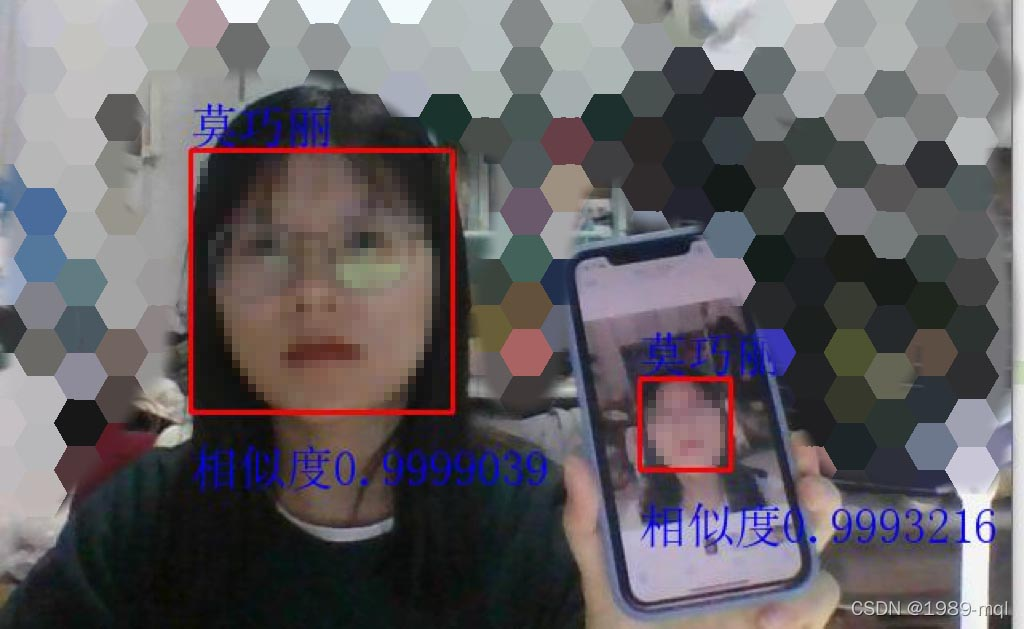﻿ 解读卷积神经网络的人脸识别_python_脚本之家
python# 解读卷积神经网络的人脸识别

## 使用环境

• python3.7
• tensorflow 2.2.0
• opencv-python 4.4.0.40
• Keras 2.4.3
• numpy 1.18.5，具体安装过程以及环境搭建省略，可借鉴网上。

## 实现步骤如下图所示### 1. 人脸数据采集与读取

1.1 数据采集

```import os
import cv2
import time
from PIL import Image

#只实现截屏的功能
global path
path='./images/'
#人脸采样,封装函数
def cy(path):
#path为保存图片的路径
#调用笔记本内置摄像头，参数为0，如果有其他的摄像头可以调整参数为1,2
cap = cv2.VideoCapture(0)
#为即将录入的脸标记一个id
face_id = input('\n 用户脸部信息录入，输入用户名字（最好用英文）：\n')
#sampleNum用来计数样本数目
count = 0

while True:
#从摄像头读取图片
count += 1
#保存图像，把灰度图片看成二维数组来检测人脸区域
#保存到相应的文件夹里
cv2.imwrite(path+str(face_id)+'.'+str(count)+'.jpg',img)
#显示图片
cv2.imshow('image',img)
#保持画面的连续。waitkey方法可以绑定按键保证画面的收放，通过q键退出摄像
k = cv2.waitKey(1)
if k == '27':
break
#或者得到800个样本后退出摄像，这里可以根据实际情况修改数据量，实际测试后800张的效果是比较理想的
elif count >= 500:
time.sleep(2)
break

#关闭摄像头，释放资源
cap.release()
cv2.destroyAllWindows()
#调用函数进行人脸采样
cy(path)```

```import cv2
import os

#对图片进行处理，输入的不是灰度图片,方便外部采集的图片进行处理
RAW_IMAGE_DIR = 'images/'
DATASET_DIR = 'hh/'
path='D:\\pythonlx\\test\\images\\'

#人脸分类器

#定义人脸大小
def save_feces(img, name,x, y, width, height):
image = img[y:y+height, x:x+width]
cv2.imwrite(name, image)

image_list = os.listdir(RAW_IMAGE_DIR) #列出文件夹下所有的目录与文件
for image_path in range(len(image_list)):
gh=path+image_list[image_path]
# print(gh)
#gray = cv2.cvtColor(image, cv2.COLOR_BGR2GRAY)
scaleFactor=1.2,
minNeighbors=5,
minSize=(5, 5), )
for (x, y, width, height) in faces:
save_feces(image, '%ss%d.jpg' % (DATASET_DIR, image_path+1), x, y - 30, width, height+30)
```

1.2 数据读取

``` #读取图片
data_x, data_y = [], []
image_list = os.listdir('mine/')
for i in range(len(image_list)):
try:
im = resize_without_deformation(im)
data_x.append(np.asarray(im, dtype = np.int8))
#定义标签
a=image_list[i].split('.')
if a=='s2':
data_y.append(0)
elif a=='s4':
data_y.append(1)
elif a=='s5':
data_y.append(2)
elif a=='s6':
data_y.append(3)
elif a=='s7':
data_y.append(4)
except IOError as e:
print(e)
except:
print('Unknown Error!')
return data_x,data_y

#读取所有图片以及标签
##查看数据每个标签的数据量
#a=raw_labels.count(0)#583
#b=raw_labels.count(1)#621
#c=raw_labels.count(2)#717
#d=raw_labels.count(3)#765
#e=raw_labels.count(4)#698

#转为浮点型
raw_images, raw_labels = np.asarray(raw_images, dtype = np.float32),np.asarray(raw_labels, dtype = np.int32)

#将标签转化为one_hot类型
ont_hot_labels = np_utils.to_categorical(raw_labels)

#划分数据集，训练集80%，测试集20%
train_input, valid_input, train_output, valid_output =train_test_split(raw_images,
ont_hot_labels,
test_size = 0.2)
#数据归一化处理
train_input /= 255.0
valid_input /= 255.0```

### 2. 图片预处理

```def resize_without_deformation(image, size = (100, 100)):
height, width, _ = image.shape
#对于长度不等的边，找到最长边
longest_edge = max(height, width)
#使用0填充边框
top, bottom, left, right = 0, 0, 0, 0
#计算短边需要增加多上像素宽度使其与长边等长
if height < longest_edge:
height_diff = longest_edge - height
top = int(height_diff // 2)
bottom = height_diff - top
elif width < longest_edge:
width_diff = longest_edge - width
left = int(width_diff // 2)
right = width_diff - left
#给图像增加边界，是图片长、宽等长，cv2.BORDER_CONSTANT指定边界颜色由value指定
image_with_border = cv2.copyMakeBorder(image, top , bottom, left, right, cv2.BORDER_CONSTANT, value = [0, 0, 0])

resized_image = cv2.resize(image_with_border, size)
#将裁剪的图片转化为灰度图
resize_image= cv2.cvtColor(resized_image,cv2.COLOR_BGR2GRAY)
#直方图均衡化
hist = cv2.equalizeHist(resize_image)
img2 = hist.reshape((100, 100, 1))
return img2```

### 3. 模型搭建与训练``` #搭建卷积神经网络，顺序模型
model = models.Sequential()

#卷积层，卷积核大小32，每个大小383,步长为1，输入的类型为（100,100,1）,1为通道,激活函数relu
input_shape = (100, 100,1),
activation='relu'))

activation='relu'))

#池化层

#Dropout层

#卷积层
strides = (1, 1),
activation = 'relu'))#5

strides = (1, 1),
activation = 'relu'))#6

#池化层

#全连接

#输出层，神经元数是标签种类数，使用sigmoid激活函数，输出最终结果

#优化模型,
#SGD----梯度下降算子
#learning_rate = 1#学习率
#decay = 1e-6#学习率衰减因子
#momentum = 0.8#冲量
#nesterov = True
#sgd_optimizer = SGD(lr = learning_rate, decay = decay,
#                    momentum = momentum, nesterov = nesterov)

#categorical_crossentropy
metrics=['accuracy'])

#输出模型参数
model.summary()

#定义数据生成器用于数据提升，其返回一个生成器对象datagen，datagen每被调用一
#次其生成一组数据（顺序生成），节省内存，其实就是python的数据生成器
datagen = ImageDataGenerator(
featurewise_center = False,             #是否使输入数据去中心化（均值为0），
samplewise_center  = False,             #是否使输入数据的每个样本均值为0
featurewise_std_normalization = False,  #是否数据标准化（输入数据除以数据集的标准差）
samplewise_std_normalization  = False,  #是否将每个样本数据除以自身的标准差
zca_whitening = False,                  #是否对输入数据施以ZCA白化
rotation_range = 20,                    #数据提升时图片随机转动的角度(范围为0～180)
width_shift_range  = 0.2,               #数据提升时图片水平偏移的幅度（单位为图片宽度的占比，0~1之间的浮点数）
height_shift_range = 0.2,               #同上，只不过这里是垂直
horizontal_flip = True,                 #是否进行随机水平翻转
vertical_flip = False)                  #是否进行随机垂直翻转

#计算整个训练样本集的数量以用于特征值归一化、ZCA白化等处理
datagen.fit(train_input)

#利用生成器开始训练模型
history=model.fit_generator(datagen.flow(train_input, train_output,batch_size = 50),
epochs = 10,
validation_data = (valid_input, valid_output))

#模型验证
print(model.evaluate(valid_input, valid_output, verbose=2))

##画出LOSS变化曲线图
plt.plot(history.history['accuracy'])
plt.plot(history.history['val_accuracy'],label='val_accuracy')
plt.xlabel('Epoch')
plt.ylabel('Accuracy')
plt.ylim([0.5,1])
plt.legend(loc='lower right')

#保存模型
MODEL_PATH = 'face_model.h5'
model.save(MODEL_PATH)```

### 4. 识别与验证

• 识别自己与自己：• 识别自己与非己：